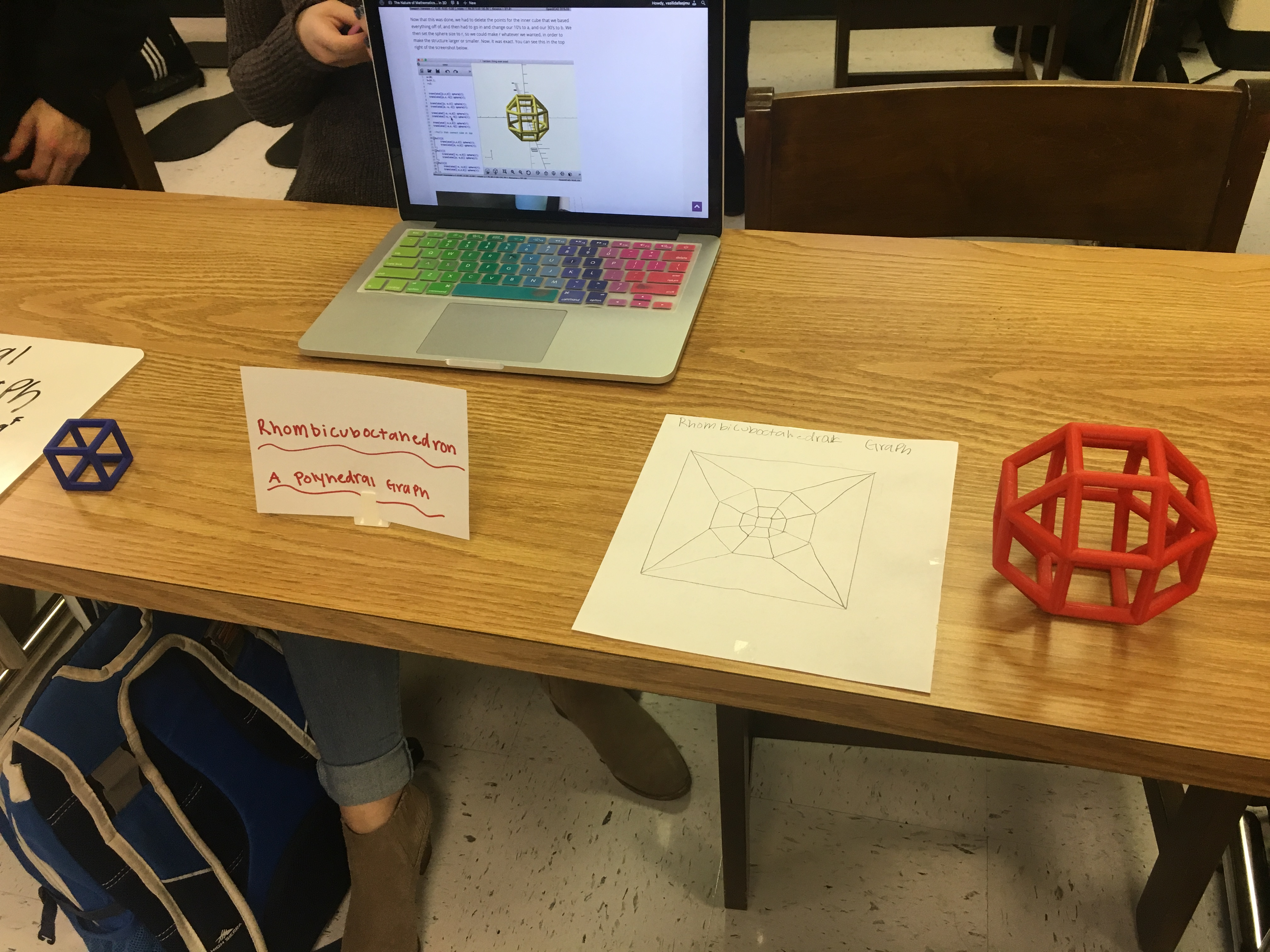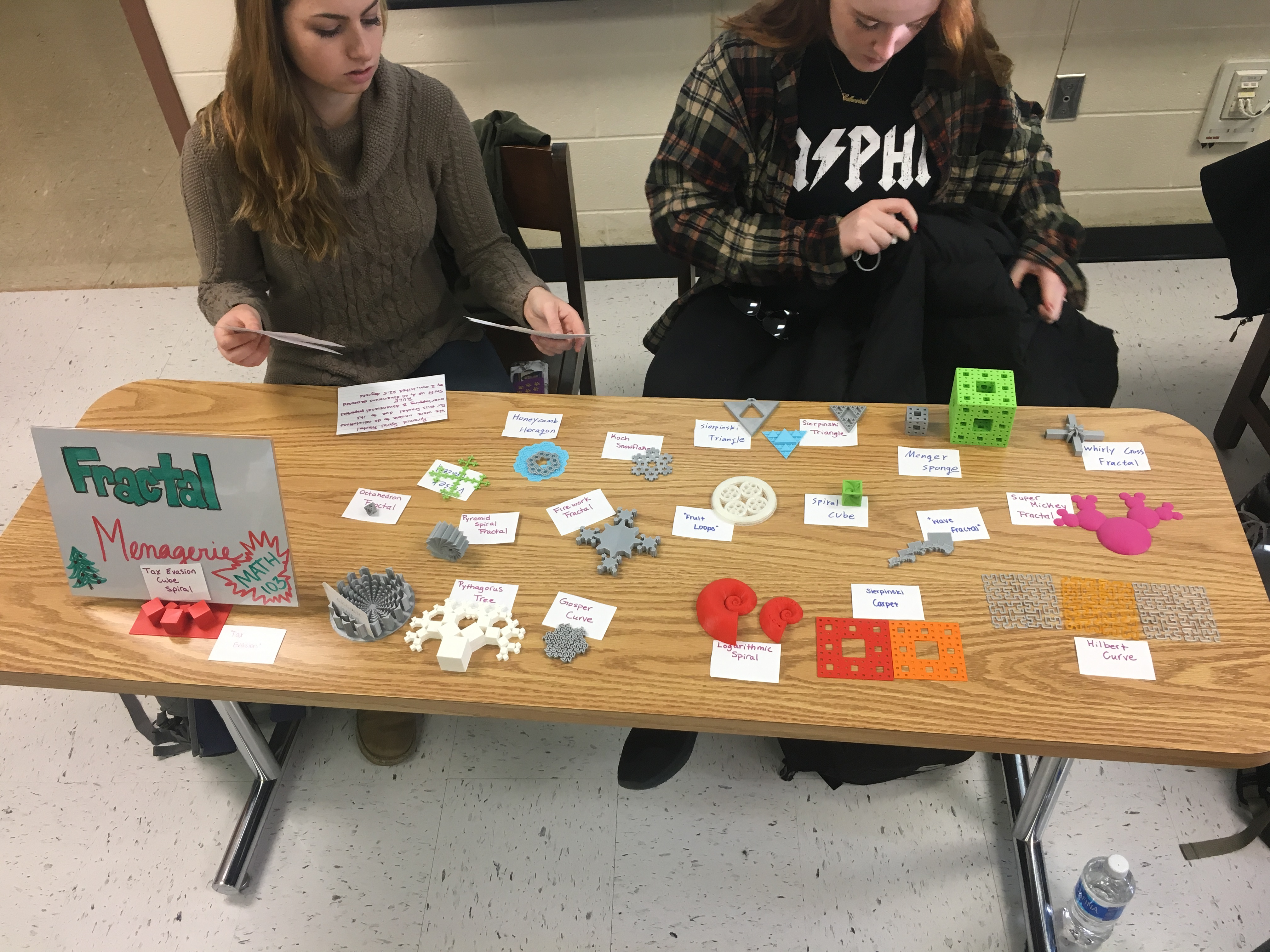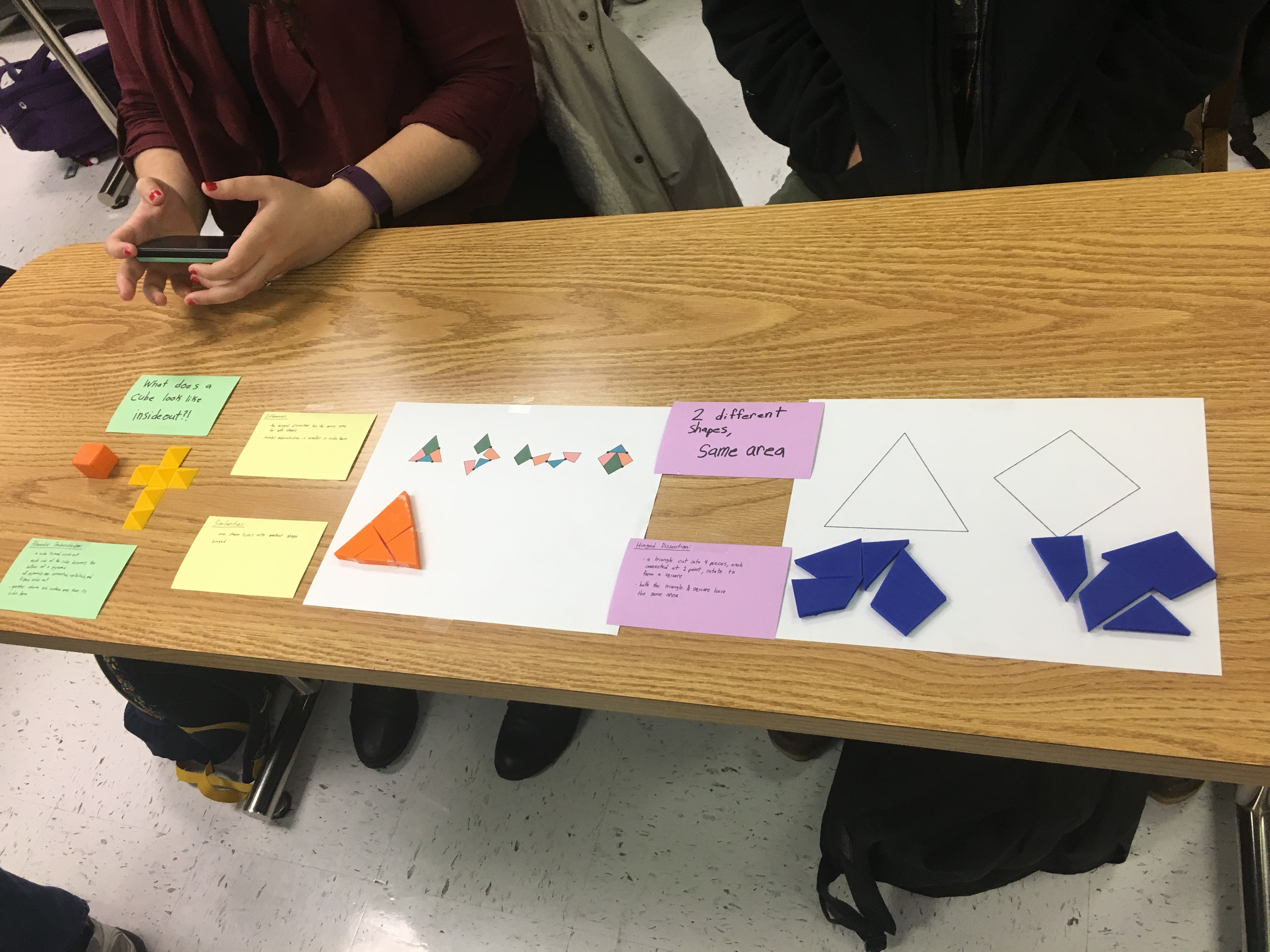# Fractal Menagerie

Cat Falvey

Yang Zhou

## Fractals

A fractal is a curve or geometric figure which parts’ have the same statistical characteristics as the whole.  Fractals follow patterns or rules that recur at progressively larger or smaller scales.  An iteration is one repetition of this rule, and fractals have many iterations or levels.  As a part of this class we were first asked to research fractals, and find ones that we found interesting.  We were then asked to come up with our own rule and create our own fractals.  This menagerie contains many of the fractals that were found and created over the course of this class.  As a part of these projects we made calculations and prints of our fractals.  Some of the calculations we made were for perimeter, area, length, and dimension.  With more complex objects these calculations were very difficult, but below we were able to illustrate more simple calculations that are easy to follow.

## Perimeter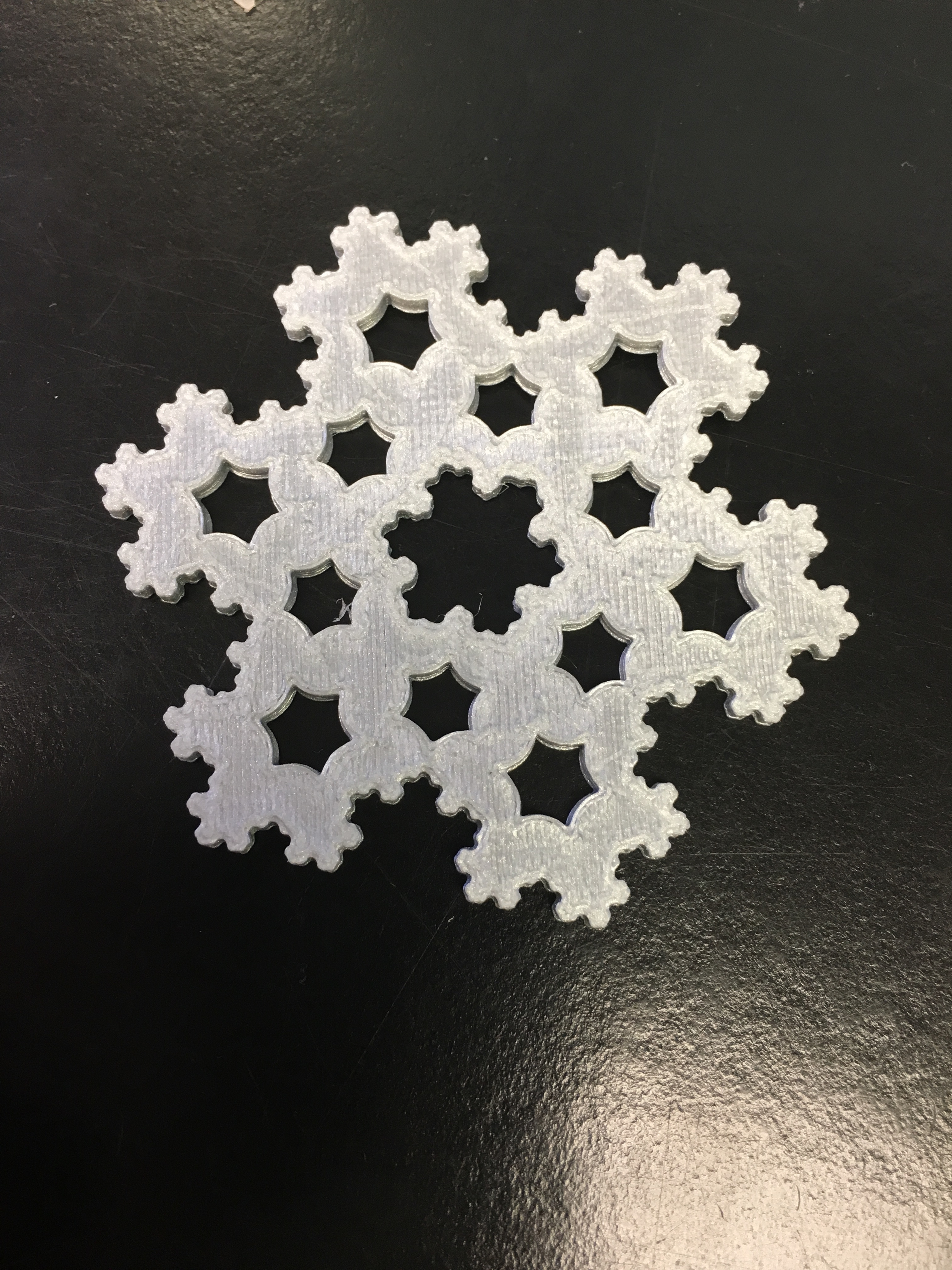The koch snowflake is one of the most famous and well documented fractals in mathematics.  It is based off of the von koch curve.  This fractal has an infinite perimeter.  We got the 4/5 value for calculations since each side of the triangle is being divided into thirds.

Level 0

P=(4/3)^0 x 3

P=1(3)=3

Level 1

P=(4/3)^1 x 3

P=(4/3) x (3/1) = 12/3

P=4

Level 2

P=(4/3)^2 x 3

P=(16/9) x (3/1) = 48/9

P=16/3

## Area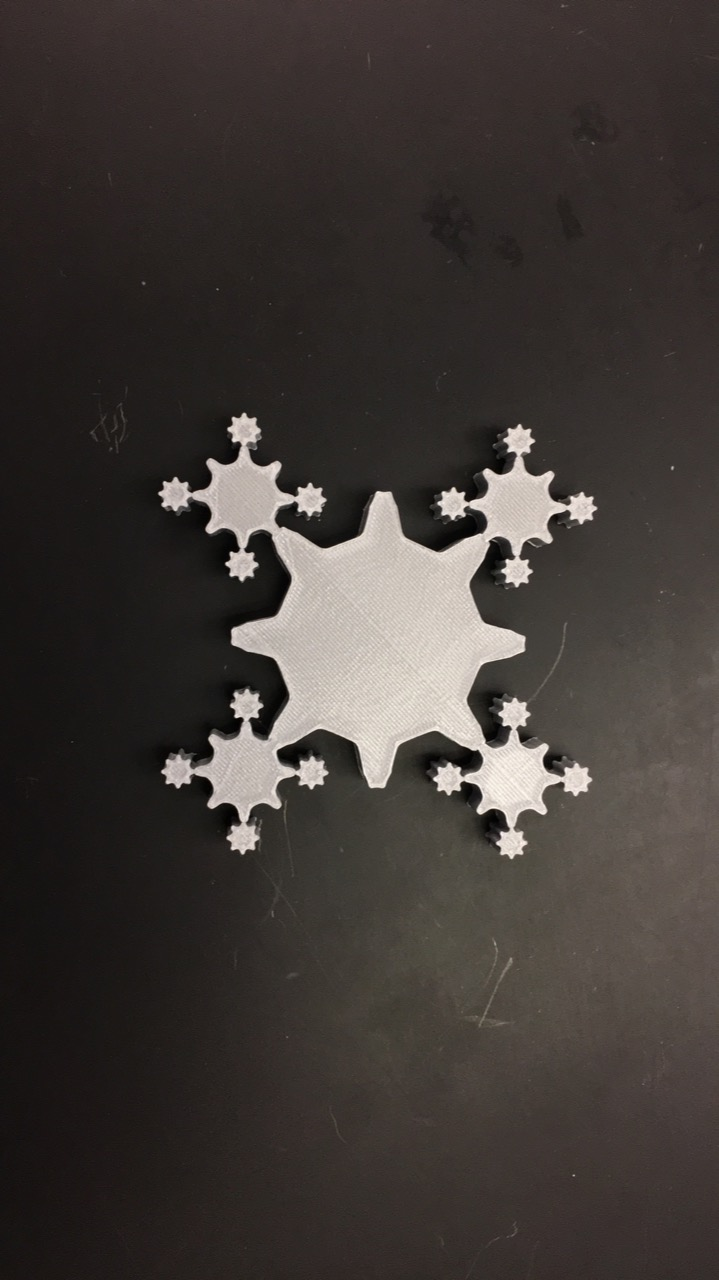The area of this fractal is finite because its decimal value only goes up slightly every iteration, so it will continue to not exceed a bounded area.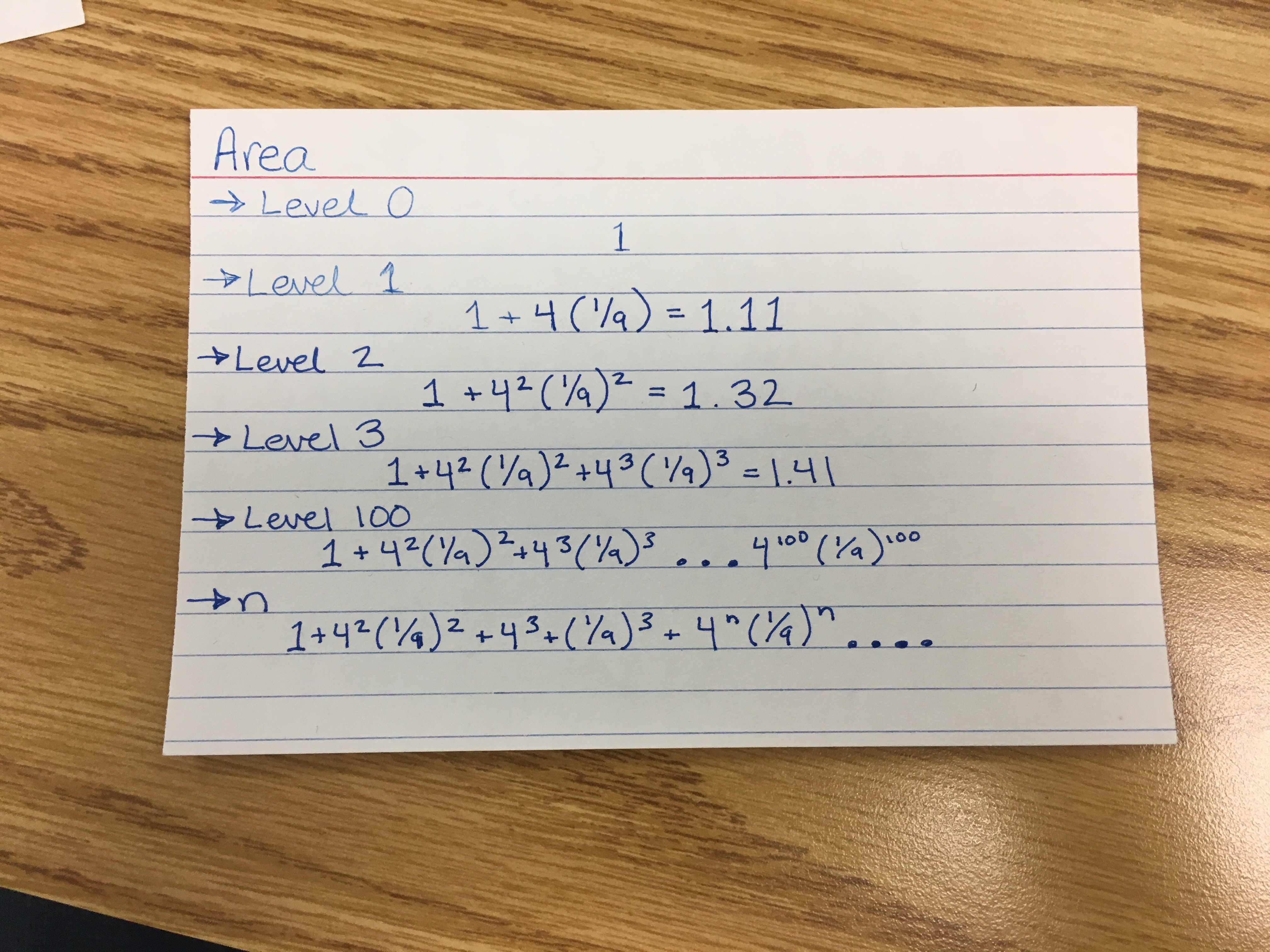## Showcase